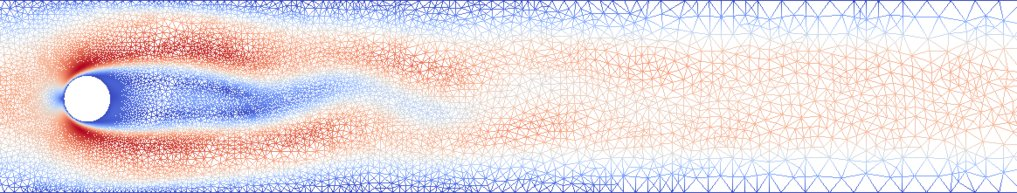Adaptive and data informed model order reduction for optimal control of complex systems

Complex systems can often be described by partial differential equations based on physical laws. In order to solve such problems on computing machines, discretization methods need to be applied which can easily lead to large-scale systems which are expensive to solve. In particular, many practical applications require a high resolution in order to reflect the system’s geometry and behavior sufficiently well. The computational barrier becomes even more serious in multi-query scenarios like optimal control. In order to decrease the computational costs, reduced-order models are introduced to replace the high-dimensional complex systems by low-order surrogates. However, it is not guaranteed automatically that reduced-order approximations deliver sufficiently accurate solutions in regards to the (unknown) true solution. First of all, the reduced-order model is constructed from a set of snapshots, which should reflect most relevant dynamical properties of the underlying system. In order to select good snapshots efficiently, we use adaptive strategies in space and time. This requires the development of e.g. residual based aposteriori error estimates for simulation and control of the considered complex systems. An example of such an adaptive space discretization for the benchmark problem of cylinder flow is shown in Figure 1.

Apart from fluid flow, we are also interested in phase field models governed by the Cahn-Hilliard equations as well as heat flow. We develop concepts to combine adaptive discretization schemes with model order reduction techniques and exploit the respective advantages.Figure 1: Laminar flow around a cylinder using adaptive finite elements Secondly, many practical applications require highly sophisticated models which are often impractical for numerical computations due to their complexity. Hence, simplifications are often considered which lead to model errors. In addition, some problem specifications (like initial or boundary conditions, problem parameters or source terms) might be unknown or uncertain (model uncertainties). In order to learn these quantities from data and enrich the information provided by the numerical models with measurement data, we utilize ideas from variational data assimilation. In particular, we aim to find a best compromise between the (reduced) model forecast and the measured data, as shown in Figure 2 for a parabolic dynamical system.